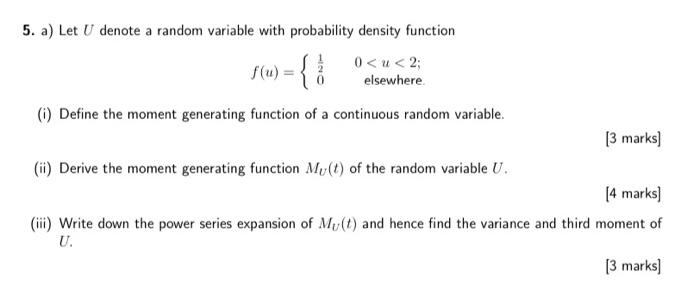# (Solved): can you solve 5a please? 5. a) Let $$U$$ denote a random variable with probability density functio ...5. a) Let $$U$$ denote a random variable with probability density function \[ f(u)=\left\{\begin{array}{rr} \frac{1}{2} & 0

We have an Answer from Expert